# Dip Coating Theory: Film Thickness

This guide is a simple introduction to the theory of dip coating which discusses the fundamentals, including the formation of the wet film, thickness evolution, and drying dynamics. It explains how processing conditions can affect the processes that occur during dip coating, and ultimately - impact the final film thickness. If you wish to learn about more specific aspects of dip coating not covered by this guide, there is a list of further reading at the end.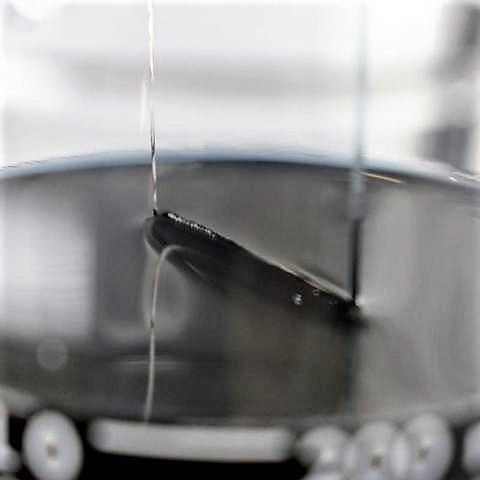A close-up view of the meniscus formed where the substrate meets the solution during dip coating.

## Overview of Dip Coating

Dip coating involves the deposition of a liquid film via the precise and controlled withdrawal of a substrate from a solution. This is typically done by using an instrument known as a 'dip coater'. There are several stages that occur during the dip coating process:

• Immersion

• Dwelling

• Withdrawal

• Drying

• Curing (Optional)

Figure 1 shows a simplified way in which the liquid film forms when a substrate undergoes the dip coating process. The substrate is lowered into a bath of solution until it is mostly or fully immersed. A short delay occurs before the substrate is withdrawn. During the withdrawal process, a thin layer of the solution remains on the surface of the substrate. Once fully withdrawn, the liquid from the film begins to evaporate and leaves behind a dry film. For certain materials, a further curing step can be performed; this forces the deposited material to undergo a chemical or physical change.

All these stages are essential in the dip coating process. However, the two critical stages for determining the properties of the deposited film are the withdrawal and drying stages.## Withdrawal and Film Formation

The withdrawal stage of the dip coating process can be simply seen as the interaction of several sets of forces. These forces can be placed into one of two categories: draining forces and entraining forces. Draining forces work to draw the liquid away from the substrate and back towards the bath. Conversely, entraining forces are those that work to retain fluid onto the substrate. The balance between these sets of forces determines the thickness of the wet film coated onto the substrate. During the withdrawal stage, the formation of the wet film can be broken into four regions (shown in Figure 2).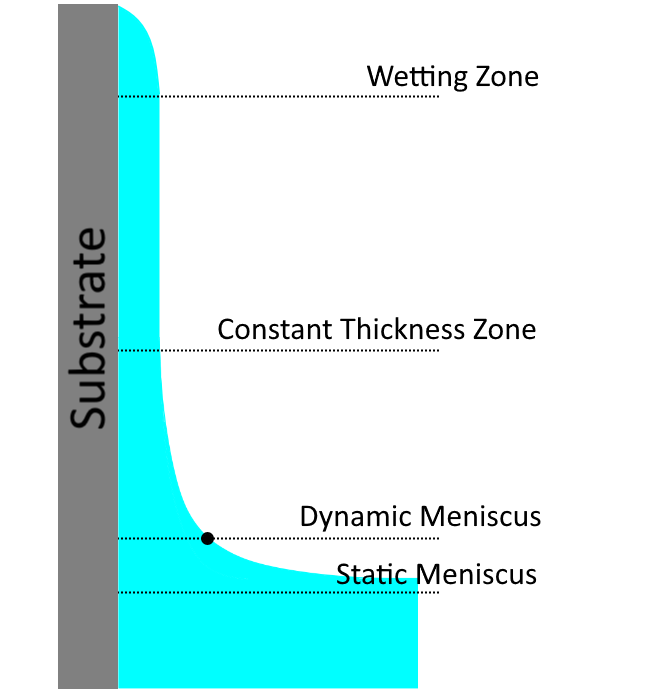Figure 2. The dip coating film formation involves four distinct regions. These are the static meniscus, the dynamic meniscus, the constant thickness zone, and the wetting zone.

These four regions are:

1. The static meniscus - Here, the shape of the meniscus is determined by the balance of the hydrostatic and capillary pressures.
2. The dynamic meniscus - This occurs around the stagnation point. The stagnation point is where the entraining forces and draining forces are in equilibrium.
3. The constant thickness zone - Here, the wet film has reached a given thickness (h0).
4. The wetting zone - This is the region where the wet film begins.

The dynamic meniscus and the flow of solution in this region determine the wet film thickness. Therefore, understanding the physics that underpins the curvature of the dynamic meniscus and the thickness of the stagnation point are important.

Figure 3 (below) shows the dynamic meniscus region. The transition between the static and dynamic meniscus occurs within the boundary layer (L). In this region, the forces from viscous flow impact the way the solution moves. Beyond the boundary layer, the draining forces are significantly greater than those of the viscous forces. In this region, it is the balance between the hydrostatic and capillary pressure that determines the meniscus.

The stagnation point occurs when the balance between the entraining forces and the draining forces are equal. It is the balance of these forces that determine the film thickness. There are three different coating regimes that are defined by which forces dominate the behaviour of the coating - these are the viscous flow, draining, and capillary regimes.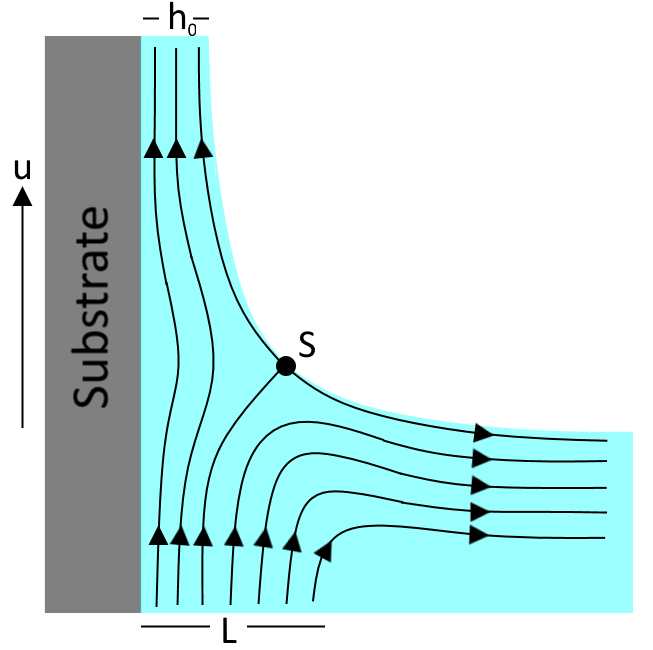Figure 3. The flow of solution is determined by the balance of entraining and draining forces. At the dynamic meniscus, the entraining forces begin to impact solution flow until they are the dominant force.

## Viscous Flow and Drainage Regime

The first coating regime is the viscous flow regime. This occurs for high velocities and viscous solutions. Here, the coating is dominated by viscous forces and gravitational attraction. In this case, the thickness of the liquid layer can be given by Equation 1.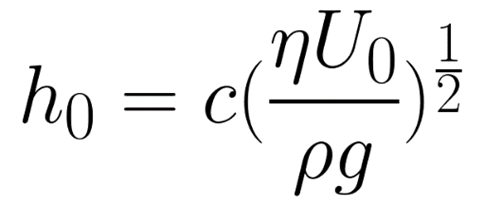Equation 1. The equation for calculating the wet film thickness for dip coating while in the viscous flow regime.

Here, the entraining force is made up of the viscous forces acting on the solution as the substrate is withdrawn. This is given by the viscosity (η) and the rate of withdrawal speed of the substrate from the solution (U0). The draining force is that of gravity, given by the density of the solution (ρ) and the gravitational constant (g). The constant (c) is related to the curvature of the dynamic meniscus. This constant is a property of the solution itself, and is strongly related to the rheological properties of the solution. For most Newtonian liquids, this constant is around 0.8.

In most situations, the withdrawal speeds or the viscosity of the solutions used will not be high enough for this approximation to be valid. When these two variables are reduced, the viscous force becomes weaker. The balance between the entraining forces and the draining force is then also dependent upon surface-tension driven movement of the solution. It is under these conditions that the coating is said to be within the drainage regime. The Landau-Levich equation (refer to Equation 2) describes the relationship between the wet film thickness and the withdrawal speed of the substrate (when surface tension is considered).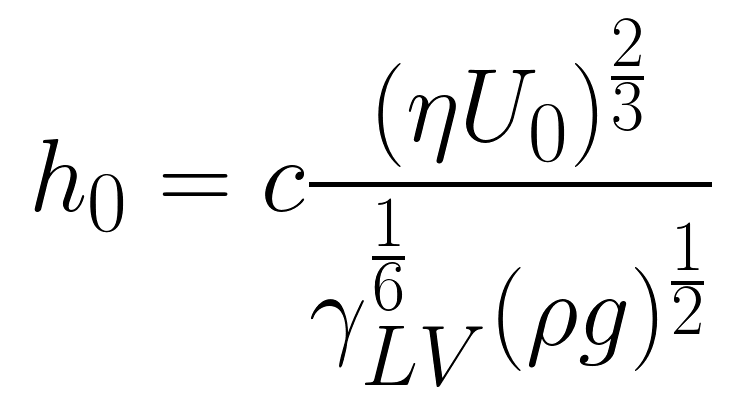Equation 2. The Landau-Levich equation is a modified form of the viscous flow equation, which takes account of surface-tension driven flow.

The Landau-Levich equation is valid until very low withdrawal speeds are considered. When the speed is reduced to lower than approximately 0.1mm.s-1, a third regime of coating occurs. This regime is known as the capillary regime. In the capillary regime, the rate at which solution is entrained onto the substrate (through viscous flow) is lower than the rate of evaporation. Therefore, the dynamics of drying are crucial to understanding the capillary regime.

## Drying Dynamics

Dip coating typically has three different stages for drying:

1. Drying front during coating
2. The constant rate period
3. The falling rate period

The simplest drying stages are the constant rate period and the falling rate period. The constant rate period occurs within the constant thickness zone (during and after coating). Here, evaporation of solvent happens at the surfaces of the wet film and occurs uniformly across it. The only exception to this is at the edges of the substrate, where the drying front occurs.

Over time, most of the solvent will be removed from the wet film until a gel-like film is formed. This is when the falling rate period occurs. In the falling rate period, the small amount of solvent left becomes trapped within the gel - and the evaporation is determined by the diffusion of solvent towards the surface.

The more complex drying stage occurs at the drying front (shown in Figure 4). The drying front appears at the interface between the wet film and substrate - the most obvious one being at the wetting zone. Due to the greater surface-area-to-volume, evaporation occurs much faster here, leading to the formation of a wet film with higher concentration. This results in the drawing of solution from the surrounding areas due to surface-tension driven effects. Once the solution at the drying front forms a dry film, a capillary force will be exerted on the solution. This causes the solution to wick into the dry film - resulting in the thickening of the deposited film.

Solution being drawn into the drying film (because of the capillary force) occurs during the capillary regime of coating. For fast enough withdrawal speeds, the rate at which the drying front recedes is significantly lower than the formation of the constant thickness zone. Therefore, the drying dynamics are dominated by the constant rate period, and the final film thickness will be dependent upon the initial wet film thickness. For slow withdrawal speeds (where the drying front recedes faster than the rate of withdrawal), the drying dynamics are dominated by the drying front.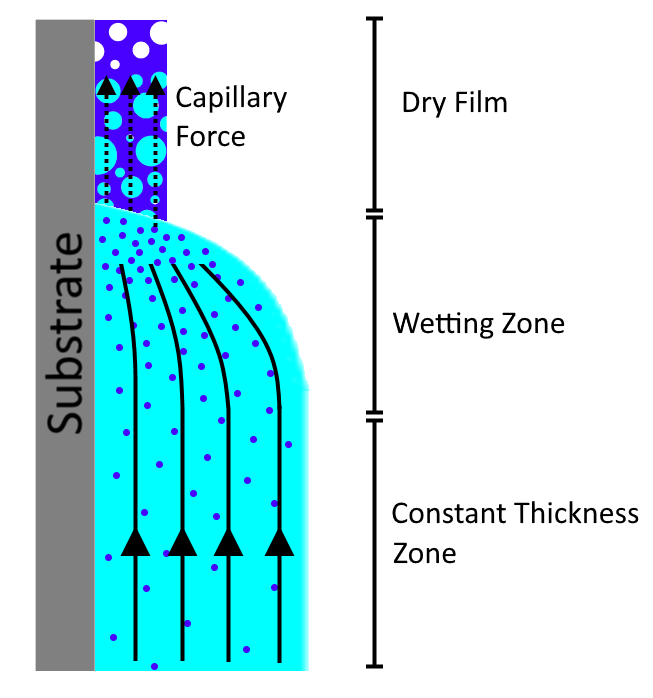Figure 4. The dynamics of drying in dip coating are controlled by the formation of concentration gradient and capillary action of the dry film.

## Capillary Regime

In the capillary regime, the wet film thickness is not considered. This is because a constant thickness zone is never truly achieved at these coating speeds. In the capillary regime, the final thickness is therefore dependent upon i) the rate of withdrawal, ii) the properties of the solution, and iii) the evaporation rate of the solvent. The dry film thickness is therefore given by Equation 3.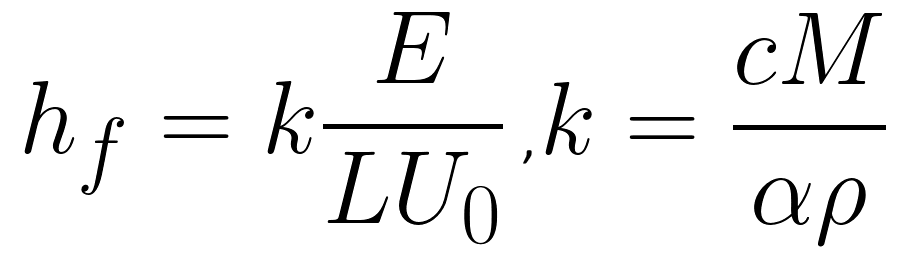Equation 3. The Capillary flow equation is dependent upon both the speed and the evaporation rate of the solvent. A constant (k) called the 'materials proportion constant' is used for the dry film properties.

Here the evaporation rate (E), the width of the coated film (L), the withdrawal rate (U0), and the materials proportion constant (K) determine the final dry film thickness (hf). K is a combination of solute, solution, and dry film properties. The constant is due to the total concentration of the solute in solution (c), the molar weight of the solute (M), the density of the solute (ρ), and the porosity of the deposited film (α).

Properties such as concentration of solute, density of solute, and molecular weight of the material have a simple and obvious impact on the dry film thickness. However, the porosity value is significantly more complex. Not only does the porosity vary the density of the film in comparison to the raw material itself - it also impacts the drying dynamics. As mentioned previously - at the drying front, at the point of contact between the dry film and the wet film, the wet film will be drawn into the dry film via capillary action. The porosity of the film also strongly influences this, determining the rate at which solution will be drawn into the dry film, the distance into the dry film it will go, and the rate at which this absorbed material will dry.

## Film Thickness vs Withdrawal Speed

The dry film thickness as a function of withdrawal speed requires both the Landau-Levich equation and the capillary regime equation to be used. Figure 5 shows an example withdrawal speed vs film thickness graph for the dip coating process. A minimum thickness for dip coating is achieved during the crossover between these two coating regimes. As the withdrawal speed goes away from the minimum in either direction, the different coating regimes dominate.

For high and low speeds, the thickness curve can be given by either the Landau-Levich equation or the capillary regime equation alone. However, for a range of coating speeds, neither equation alone can give accurate values for the coating thickness. This occurs at the absolute minimum for coating thickness. To calculate the minimum thickness, you need an equation that unifies both the Landau-Levich and the capillary regime equation. This will be discussed in the section below.Figure 5. Both the capillary regime equation and the Landau-Levich equation can be used to determine the film thickness. At the crossover between the two regions, both equation must be taken into account.

## Determining the Minimum Film Thickness

To determine the minimum thickness, both the equations governing the thickness during the drainage regime and the capillary regime must be combined. The first step in this is modifying Equation 2 to relate the wet film thickness to the dry film thickness. This can be done simply by including the 'materials proportion' constant into the equation.

Summing up both equations gives you Equation 4. Here, the first term in the bracket is related to the capillary regime (Equation 3) while the second term is related to the drainage regime (Equation 2). The constants seen in Equation 2 have been rolled into a universal solution constant (D).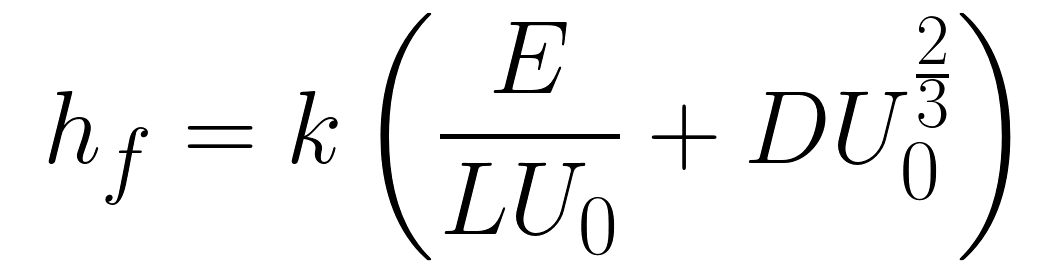Equation 4. The equation for film thickness gives information on the final dry film thickness and includes the contribution from the capillary and drainage regimes.

From this equation, it is possible to determine the smallest film thickness by differentiating the thickness with respect to withdrawal rate. By setting this derivative at 0 (which is the gradient at the inflection point of the minimum), you get Equation 5.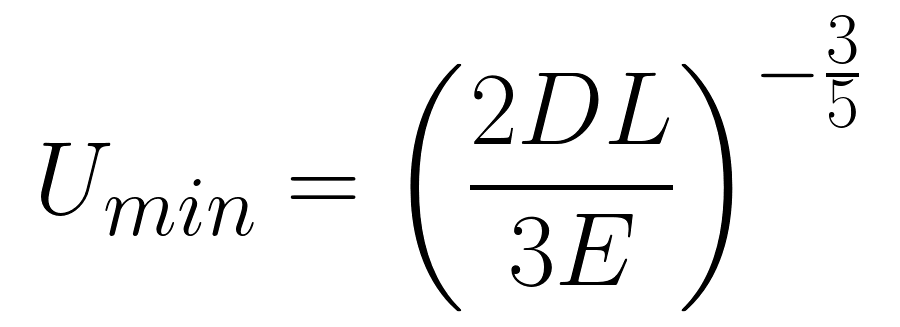Equation 5. The minimum thickness for dip coating can be found by taking the differential of the thickness equation and determining where the slope of the graph becomes zero.

Although this equation gives a close approximation to the actual film thickness achieved, there are several factors that have been ignored when deriving the equations that make it. These mainly include parameters that can vary over time (due to effects such as Marangoni Flow, variable evaporation rates, air flow at the surface, etc).## Conclusion

Dip coating requires a minimum of four unique steps to be performed -  immersion, dwelling, withdrawal, and drying. The final film thickness is determined by the interplay between the entraining forces, draining forces, and the drying of the film. The films are formed in one of three regimes (viscous flow, drainage, and capillary), with transitions between them occurring at varying values of withdrawal speed and solution viscosity.

The combination of the three coating regimes ultimately determine the 'thickness vs withdrawal speed' behaviour for a thin film. By summing the contributions from the drainage regime and capillary regime, it is possible to obtain an equation that explains the thickness withdrawal speed relationship over a wide range of speeds. It also allows us to determine the minimum possible thickness that can be coated for a solution.

Although these equations are a good approximation for the actual values, many other contributing factors to the final film formation have not been considered. These include air flow, viscosity and concentration gradients, thermal gradients, and Marangoni flow.

If you are looking to start using dip coating in your experiments, then the Ossila Dip Coater is perfect for you!

For more information on different aspects of dip coating, we recommend the following bodies of work:

Ossila: Dip Coating Theory: Film Properties (Coming Soon)

Ossila: Dip Coating Guide: Coating Parameters (Coming Soon)

Review Article: Withdrawing a solid from a bath: how much liquid is coated? E. Rio, & F. Boulogne.

Book: Sol-Gel Technologies for Glass Producers and Users. M. A. Aegerter, & M. Mennig.

Book: Chemical Solution Deposition of Functional Metal Oxide Thin Films. T. Schneller, R. Waser, M. Kosec, & D. Payne.

To the best of our knowledge, the technical information provided here is accurate. However, Ossila assume no liability for the accuracy of this information. The values provided here are typical at the time of manufacture and may vary over time and from batch to batch.